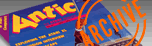`ANTIC VOL. 1, NO. 5 / DECEMBER 1982`

# Dragon Smoke Word's Worth

By George Firedrake and Bob Albrecht

DragonSmoke poses problems for you to ponder, questions for you to answer, programs for you to write, and whatever other mischief we might cunningly contrive. As time goes on, and as issue follows issue, we will solve some of the problems we create. Better yet, you will solve them.

## ASCII LAND

Deep down inside the computer, each keyboard character has its very own numeric code, called an ASCII code. ASCII means American Standard Code for Information Interchange. This is only the beginning of life for a keyboard character. Of course, every character wants to be part of TRON.

• The ASCII code for A is 65.
• The ASCII code for B is 66.
• The ASCII code for C is 67.
• Guess. What is the ASCII code for D?
• Guess. What is the ASCII code for Z?

The ASCII codes for upper case letters A to Z are the numbers 65 to 90.

Atari BASIC provides a built-in function, called ASC, which gives the ASCII code for any character. Clear the screen and try these.

You type: PRINT ASC("A") It prints: 65
You type: PRINT ASC("B") It prints: 66
You type: PRINT ASC("Z") It prints: 90

REMEMBER: If a character is an upper case letter, A to Z, its ASCII code is a number, 65 to 90. A character whose ASCII code is less than 65 or more than 90 is not an upper case letter A to Z. You might use this fact in a program we suggest near the end of this article.

## WORD S WORTH # 1

Assign a number to each letter of the alphabet, A to Z, as follows.

```	A= 1	B = 2	C= 3	D= 4	E = 5	F = 6
G = 7	H = 8	I = 9	J =10	K =11	L =12
M=13	N =14	0=15	P =16	Q = 17	R =18
S =19	T =20	U =21	V =23	W=23	X =24
Y =25   Z =26
```
We call these letter scores (LS). The letter score of A is 1; the letter score (LS) of B is 2; the letter score (LS) of C is 3; and so onŃthe letter score of Z is 26. A Word's Worth (WW) is obtained by adding the letter scores of the letters in a word. For example, HOBBIT is worth 56, DRAGON is worth 59, and WIZARD is worth 81. The Word's Worth (WW) is the sum of the Letter Scores (LS).

Now we will ask you some questions. To answer these questions, you must find a word in a dictionary. There are many dictionaries, so let's all use the same one. The abridged American Heritage Dictionary has 55,000 words, and you can buy a copy for \$3.95 at most any bookstore.

You people with ten-pound dictionaries may also respond, but please tell us which dictionary you used, including its most recent copyright date. If you come up with an interesting answer, we might mention it in a future issue.

Okay, with dictionary in hand, try one or more of these questions.

1. How many three-letter words have a Word's Worth less than 100?

2. What three-letter word has the smallest Word's Worth?

3. What three-letter word has the largest Word's Worth?

4. In the dictionary we selected, what word has the largest Word's Worth?

5. What is the most interesting three letter word? By "interesting," we mean what word, together with its Word's Worth, is most interesting?

6. What words have a WW of 12?

7. What words have a WW of 123? And so on.

8. In our chosen dictionary, send us words whose Word's Worths are prime numbers less than 100. For each prime number, send us a word.

EXTRA CHALLENGE: As much as possible, let each word be related in some way to the prime number.

9. Send us "odd" words whose Word's Worth is an odd number and "even" words whose Word's Worth is even.

10. Add to your fun (and ours) by sending in new Word's Worth ideas.

Word's Worth is an example of a class of questions and problems in which your ATARI can do the work, while you do the thinking. You can write a program to compute the Word's Worth of any word, or string of letters, that you type in.

We challenge you to write a program to compute answers for this month's Word's Worth questions. We want you to write a program to:

• Accept a "word" (any string).

• Compute Word's Worth #1 using all the letters in the string. Throw away anything not a letter.

• Print the Word's Worth #1 of the string of letters.

Next time, we will show you our Word's Worth #1 program. We will also tell you about Word's Worth #2. In the meantime, if you want to reach us, write to: George and Bob, P.O. Box 310, Menlo Park, CA 94025. If you want a reply, enclose a self-addressed, stamped envelope (SASE).

Bob Albrecht is the author of the book Atari BASIC, that accompanies the BASIC catridge. He has been involved in computer writing and education for 20 years, and is a coowner of Dymax, a publishing company in Menlo Park, CA. George Firedrake is a dragon.